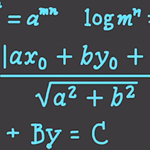# Precalculus 9 : Logarithm EquationsOver these next few Precalculus videos you will be 100% prepared to learn Calculus.

In this video I’ll cover : Logarithmic Expressions, Common Logarithm, Natural Logarithm, Exponents vs. Logarithms, Conversion, Negative Exponents, Adding Logarithms, Subtracting Logarithms, Logarithm Equality and more. Video on Logarithms & Euler’s Number, Conic Sections Circles, Conic Sections Parabolas,Conic Sections Ellipses